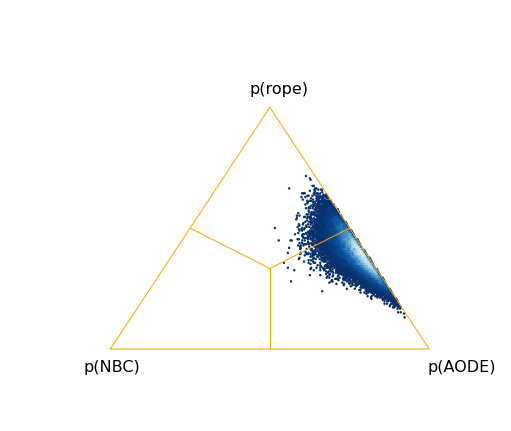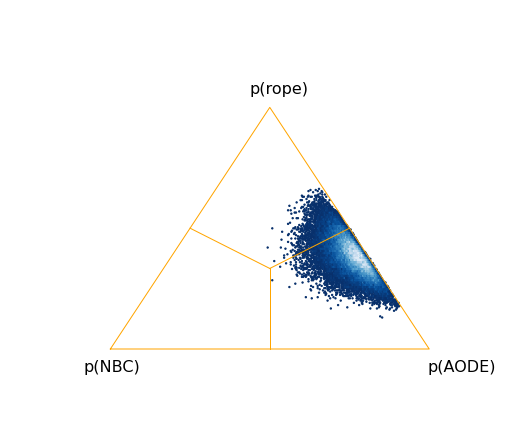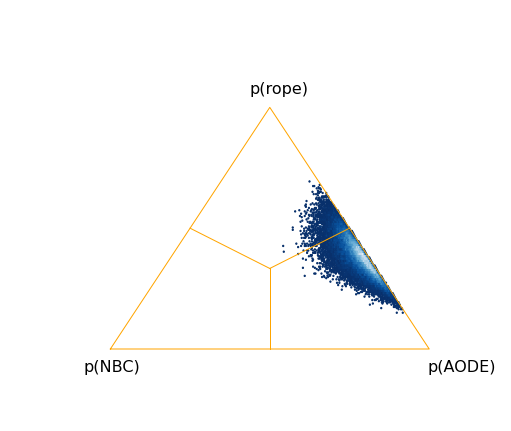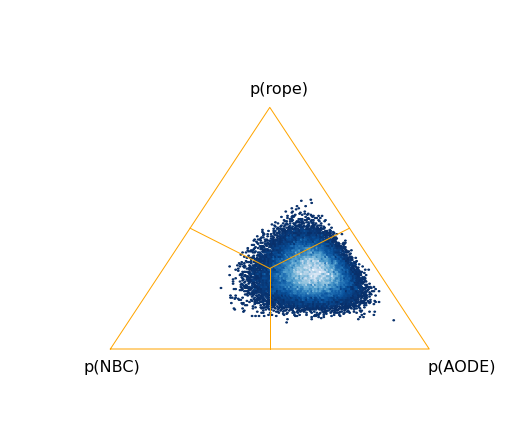# Bayesian Signed-Rank Test for comparing algorithms in machine learning

This post is about Bayesian nonparametric tests for comparing algorithms in ML. This time we will discuss about Python module signrank in bayesiantests (see our GitHub repository). It computes the Bayesian equivalent of the Wilcoxon signed-rank test. It return probabilities that, based on the measured performance, one model is better than another or vice versa or they are within the region of practical equivalence.

This notebook demonstrates the use of the module. You can download the notebook from GitHub as well.

We will load the classification accuracies of the naive Bayesian classifier and AODE on 54 UCI datasets from the file Data/accuracy_nbc_aode.csv. For simplicity, we will skip the header row and the column with data set names.

In :
import numpy as np
scores = np.loadtxt('Data/accuracy_nbc_aode.csv', delimiter=',', skiprows=1, usecols=(1, 2))
names = ("NBC", "AODE")


Functions in the module accept the following arguments.

• x: a 2-d array with scores of two models (each row corresponding to a data set) or a vector of differences.
• rope: the region of practical equivalence. We consider two classifiers equivalent if the difference in their performance is smaller than rope.
• prior_strength: the prior strength for the Dirichlet distribution. Default is 0.6.
• prior_place: the region into which the prior is placed. Default is bayesiantests.ROPE, the other options are bayesiantests.LEFT and bayesiantests.RIGHT.
• nsamples: the number of Monte Carlo samples used to approximate the posterior.
• names: the names of the two classifiers; if x is a vector of differences, positive values mean that the second (right) model had a higher score.

### Summarizing probabilities

Function signrank(x, rope, prior_strength=0.6, prior_place=ROPE, nsamples=50000, verbose=False, names=('C1', 'C2')) computes the Bayesian signed-rank test and returns the probabilities that the difference (the score of the first classifier minus the score of the first) is negative, within rope or positive.

In :
import bayesiantests as bt
left, within, right = bt.signrank(scores, rope=0.01)
print(left, within, right)

0.0 0.12244 0.87756


The first value (left) is the probability that the first classifier (the left column of x) has a higher score than the second (or that the differences are negative, if x is given as a vector).

In the above case, the right (AODE) performs worse than naive Bayes with a probability of 0.88, and they are practically equivalent with a probability of 0.12.

If we add arguments verbose and names, the function also prints out the probabilities.

In :
left, within, right = bt.signrank(scores, rope=0.01, verbose=True, names=names)

P(NBC > AODE) = 0.0, P(rope) = 0.1232, P(AODE > NBC) = 0.8768


The posterior distribution can be plotted out:

1. using the function signrank_MC(x, rope, prior_strength=1, prior_place=ROPE, nsamples=50000) we generate the samples of the posterior
2. using the function plot_posterior(samples,names=('C1', 'C2')) we then plot the posterior in the probability simplex
In :
%matplotlib inline
import matplotlib.pyplot as plt

samples = bt.signrank_MC(scores, rope=0.01)

fig = bt.plot_posterior(samples,names)

plt.show()### Checking sensitivity to the prior

To check the effect of the prior, let us a put a greater prior on the left.

In :
samples = bt.signrank_MC(scores, rope=0.01,  prior_strength=0.6, prior_place=bt.LEFT)
fig = bt.plot_posterior(samples,names)
plt.show()… and on the right

In :
samples = bt.signrank_MC(scores, rope=0.01,  prior_strength=0.6, prior_place=bt.RIGHT)
fig = bt.plot_posterior(samples,names)
plt.show()The prior with a strength of 1 has negligible effect. Only a much stronger prior on the left would shift the probabilities toward NBC:

In :
samples = bt.signrank_MC(scores, rope=0.01,  prior_strength=6, prior_place=bt.LEFT)
fig = bt.plot_posterior(samples,names)
plt.show()## Auxiliary functions

The function signrank_MC(x, rope, prior_strength=0.6, prior_place=ROPE, nsamples=50000) computes the posterior for the given input parameters. The result is returned as a 2d-array with nsamples rows and three columns representing the probabilities $p(-\infty, rope), p[-rope, rope], p(rope, \infty)$. Call signrank_MC directly to obtain a sample of the posterior.

The posterior is plotted by plot_simplex(points, names=('C1', 'C2')), where points is a sample returned by signrank_MC.

@ARTICLE{bayesiantests2016, author = {{Benavoli}, A. and {Corani}, G. and {Demsar}, J. and {Zaffalon}, M.}, title = "{Time for a change: a tutorial for comparing multiple classifiers through Bayesian analysis}", journal = {ArXiv e-prints}, archivePrefix = "arXiv", eprint = {1606.04316}, url={https://arxiv.org/abs/1606.04316}, year = 2016, month = jun }

@inproceedings{benavoli2014a, title = {A {B}ayesian {W}ilcoxon signed-rank test based on the {D}irichlet process}, booktitle = {Proceedings of the 30th International Conference on Machine Learning ({ICML} 2014)}, author = {Benavoli, A. and Mangili, F. and Corani, G. and Zaffalon, M. and Ruggeri, F.}, pages = {1--9}, year = {2014}, url = {http://www.idsia.ch/~alessio/benavoli2014a.pdf} }

This site uses Akismet to reduce spam. Learn how your comment data is processed.